﻿ 螺旋六角para-链的最大匹配的强迫数

# 螺旋六角para-链的最大匹配的强迫数The Forcing Number of Maximum Matching in Spiro Hexagonal Para-Chain

Abstract: Let G be a graph with a maximum matching M. A subset S ⊆ M is called a forcing set of M if S is con-tained in only one maximum matching M of G. A forcing set of M with minimum cardinality is the minimum forcing set of M and its cardinality is called the forcing number of M, denoted by fm(G, M). The minimum forcing number of all maximum matchings in G is called the forcing number of G, denoted by fm(G). In this paper, we obtain the forcing number of maximum matching in spiro hex-agonal para-chain.

1. 引言

2. 螺旋六角para-链的最大匹配的强迫数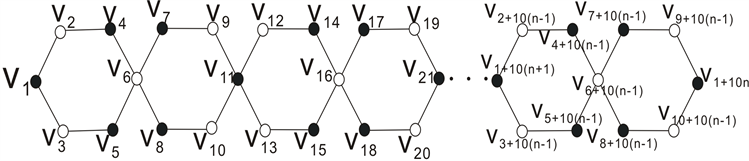Figure 1. The spiro hexagonal para-chain graph G with 2n hexagons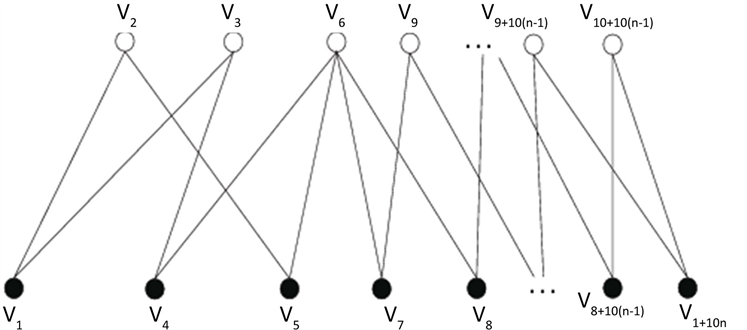Figure 2. Bipartite graph G = (A, B)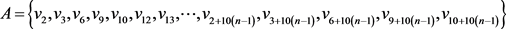B部分的点集记为：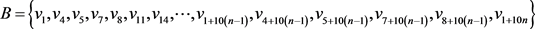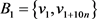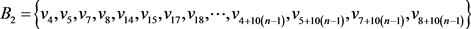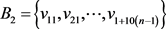Randić, M. and Klein, D.J. (1985) Kekulé Valence Structures Revisited, Innate Degrees of Freedom of π-Electron Couplings. In: Trinajstić N., Eds., Mathematical and Computational Concepts in Chemistry, Wiley, New York, 274-282.

 Harary, F., Klein, D.J. and Živković, T.P. (1991) Graphical Properties of Polyhexes: Perfect Matching Vector and Forcing. Journal of Mathematical Chemistry, 6, 295-306.
https://doi.org/10.1007/BF01192587

 Vukičević, D. and Sedlar, J. (2004) Total Forcing Number of the Tri-angular Grid. Mathematical Communications, 9, 169-179.

 Xu, S.-J., Zhang, H.-P. and Cai, J.-Z. (2015) Complete Forcing Numbers of Catacondensed Hexagonal Systems. Journal of Combinatorial Optimization, 29, 803-814.
https://doi.org/10.1007/s10878-013-9624-x

 Lovász, L. and Plummer, M.D. (1986) Matching Theory. Akademiai Kiado, Amsterdam, North-Holland.

 Vukičević, D., Zhao, S., Sedlar, J., Xu, S.-J. and Došlić, T. (2018) Global Forcing Number for Maximal Matchings. Discrete Mathematics, 341, 801-809.
https://doi.org/10.1016/j.disc.2017.12.002

 Zhong, L. (2012) The Harmonic Index on Unicyclic Graphs. Ars Combinatoria, 104, 261-269.

 Yarahmadi, Z., Došlić, T. and Moadi, S. (2013) Chain Hexagonal Cacti: Extremal with Respect to the Eccentric Connectivity Index. Iranian Journal of Mathematical Chemistry, 4, 123-126.

 王洪伟. 二部图的匹配强迫数[D]: [博士学位论文]. 兰州: 兰州大学, 2008.

Top